# Work and energy (Class 9 CBSE)

Notes Assignment NCERT Solutions

## Introduction

• In our everyday life we use terms like work and energy.
• Term work is generally used in context to any kind of activity requiring physical or mental effort.
• When we push or pull a heavy load or lift it above the floor then we are doing work, but a man carrying heavy load and standing still is not doing any work according to scientific definition of work.
• Another term we often use is energy. Energy is usually associated with work done in the sense that a person feeling very energetic is capable of doing lot of work.
• This way energy is defined to be as capacity of doing work.
• There are many forms of energy like chemical energy, mechanical energy, electrical energy, heat energy etc. These forms of energies can be used in number of ways.
• One form of energy can be converted into another form of energy.
• In this chapter we will study about work, relation between work and energy, conservation of energy etc.

## Work

• We already know that work is said to be done when a force produces motion.
• So we can formally define work done as
Work done by force acting on an object is equal to the product of force and the displacement of the object in the direction of the force.
• Work done is defined in such a way that it involves both force applied on the body and the displacement of the body.
• Consider the figure given below where a block is placed on a frictionless horizontal floor. This block is acted upon by a constant force F. Action of this force is to move the body through a distance d in a straight line in the direction of force.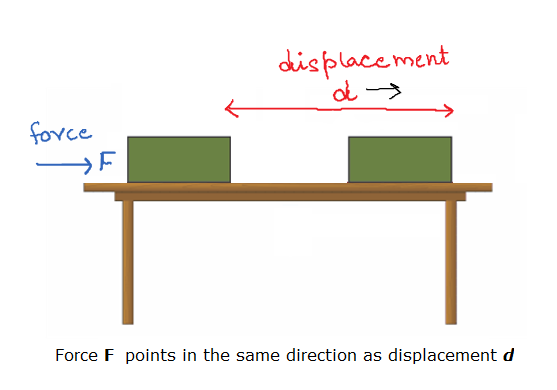• Now, work done by this force is equal to the product of the magnitude of applied force and the distance through which the body moves. Mathematically,
W=Fd
• Here both force and displacement have magnitude as well as directions. So both of these quantities are vectors. Work, which is the product of force and displacement, has only magnitude and no direction. So it is a scalar quantity.
• If F = 1 N and d = 1 m then the work done by the force will be 1 N m.
• Here the unit of work is Newton meter (N m) or joule (J). Thus 1 J is the amount of work done on an object when a force of 1 N displaces it by 1 m along the line of action of the force.
• The work done by a force can be either positive or negative or zero.

### Positive Work

•  If a force displaces the object in its direction, then the work done is positive
So, W=Fd
The example of this kind of work done is motion of ball falling towards ground where displacement of ball is in the direction of force of gravity.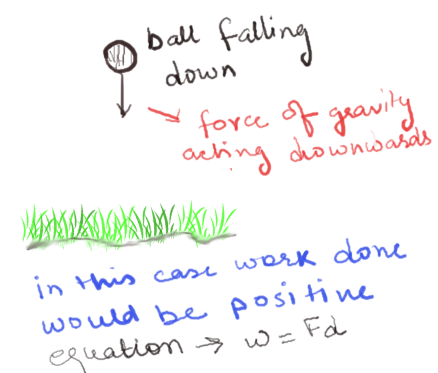• ### Negative work

• If the force and the displacement are in opposite directions, then the work is said to be negative. For example if a ball is thrown in upwards direction, its displacement would be in upwards direction but the force due to earth’s gravity is in the downward direction.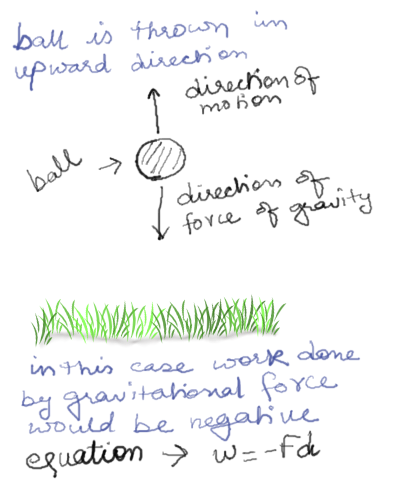So here in this case gravity is doing negative work when you throw the ball upwards. Hence the work done by gravitational force is negative. Mathematically when displacement is opposite to the force work done is given by
• Negative work just means that the force and the displacement act in opposite directions.

### Case of zero work done

• If the directions of force and the displacement are perpendicular to each other, the work done by the force on the object is zero.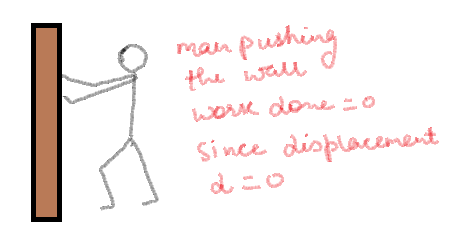For example, when we push hard against a wall, the force we are exerting on the wall does no work, because in this case the displacement of the wall is d = 0. However, in this process, our muscles are using our internal energy and as a result we get tired.

### Displacement at an angle to the force

• To understand this let us consider a block of mass m sliding on an inclined surface as shown below in the figure.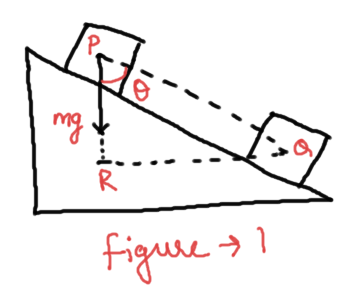• Now the object moves along the inclined surface from point P to Q. So its displacement would be PQ. The force acting on this block due to force of gravity would be
$F = mg$
which acts in vertically downwards direction.
• Now here our problem is to find the work done by the gravitational force in moving the block from point P to point Q.
• When the block moves from point P to Q, it comes down through a height PR. So here we can also say that the object is displaced in the vertically downwards direction through a distance PR and at the same time it is also displaced horizontally through a distance RQ. Since the displacement in the direction of force is PR , the work done would be
$W = mg(PR) = mg(PQcos\theta )$
• If displacement d of any object makes an angle $\theta$ with the force $F$ acting on it as shown below in the figure , then the work done by the force is
$W = Fd\cos \theta$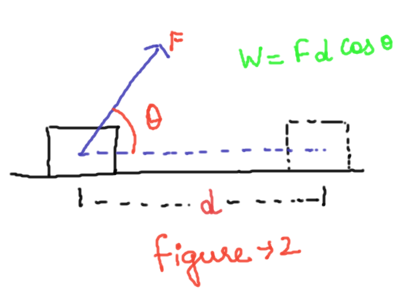• Now again consider the figure 1 where $PQ$ is the actual displacement but here you must note that $PQcos\theta$ is the displacement of the block in the direction of force which is along $PR$. So we can say that $PQcos\theta$ is the component of displacement $PQ$ along $PR$.
• So, general equation of work done would be
Work=force × component of displacement along the direction of force

Reference Books for class 9 science

Given below are the links of some of the reference books for class 9 science.

These books by S.Chand Publications are detailed in their content and are must have books for class 9 students. Some other books that might be worth considering are
1. Oswaal CBSE Question Bank Class 9 Science Chapterwise & Topicwise We all know importance of practicing what we have studied. This book is perfect for practicing what you have learned and studied in the subject.
2. Pearson Foundation Series (IIT-JEE/NEET) Physics, Chemistry, Maths & Biology for Class 9 (Main Books) | PCMB Combo These Foundation books would be helpful for students who want to prepare for JEE/NEET exams. Only buy them if you are up for some challenge and have time to study extra topics. These might be tough for you and you might need extra help in studying these books.
3. Foundation Science Physics for Class - 9 by H.C. Verma This is one of my favorite Physics book for class 9. Most of the book is within the limits of CBSE syllabus. It might overwhelm you with its language but I feel if you can understand the content is authentic with plenty of problems to solve.

You can use above books for extra knowledge and practicing different questions.

Note to our visitors :-

Thanks for visiting our website.